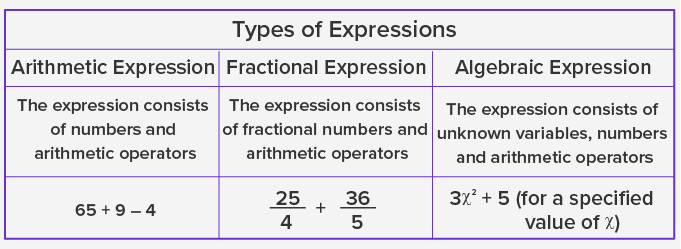# Expression - Definition with Examples

The Complete K-5 Math Learning Program Built for Your Child

• 30 Million Kids

Loved by kids and parent worldwide

• 50,000 Schools

Trusted by teachers across schools

• Comprehensive Curriculum

Aligned to Common Core

## What is an Expression?

An expression is a sentence with a minimum of two numbers and at least one math operation. This math operation can be addition, subtraction, multiplication, and division. The structure of an expression is:

Expression = (Number, Math Operator, Number)

For example

= 7 + 9

= 23 × 4

= 37 – 6

= 25 + 9 – 4 ÷ 2

In all the given expressions, a math operator is used between the two numbers.

A math expression is different from a math equation. An equation will always use an equivalent (=) operator between two math expressions.

For example,

= 25 + 7 = 64 ÷ 2

= 20 × 5 = 102

The structure of defining math expression advances in different grades. In early grades, children are expected to write math expressions using numbers and operators. Later on, words help students to form a math expression.

Let’s consider a word problem.

Tom has to fill a box with oranges and apples. The number of apples should be 5 more than oranges. Tom picks 3 oranges each time and repeats it 5 times. Count the total number of oranges and apples.

To solve this, formulate the math expressions as follows:

= Number of oranges = 3 × 5

= Number of oranges = 15

Number of apples = Number of oranges + 5

= Number of apples = 15 + 5

= Number of apples = 20

Total number of fruits = Number of oranges + Number of apples

Third math expression will be:

= 15 + 20

= 35Application

The knowledge of applying math operations on numbers is the first step towards building basic arithmetic reasoning and logic in children. Formulation of math expressions using the respective skill lays a strong foundation to learn algebra and translate real-life problems in suitable mathematical models.

 Fun Facts Each statistical test and model uses a set of mathematical expressions to analyze quantitative data Computers were invented to primarily compute long math expressions within a fraction of second

Won Numerous Awards & Honors